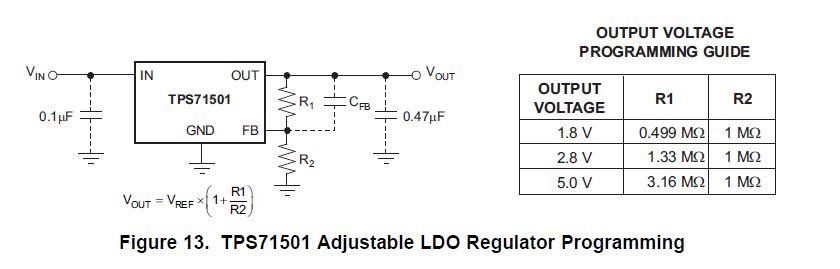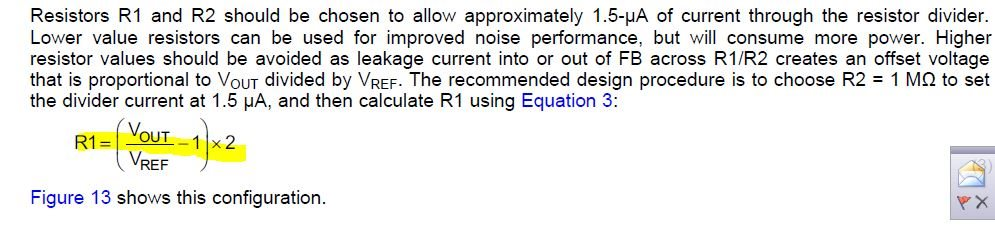If you have a related question, please click the "Ask a related question" button in the top right corner. The newly created question will be automatically linked to this question.

# TPS715: Output Voltage Programming

Part Number: TPS715

Hi Team,

I'm using the TPS71501 generate a 5-V output from a 12-V supply and the load current is about 5 mA.

When calculating the resistances of the resistor divider, I found something that contradicts each other.

1. Using the table in Figure 13, R1 and R2 should be 3.16 Mohm and 1 Mohm, respectively.2. Using Equation (3) yields R1 = 6.29 Mohm when R2 = 1 Mohm.Since the two results are quite different, could you please clarify which we should follow?

Thanks!

Roy Hsu

• Hi Roy,

It looks like there is a slight typo in the second equation. The second Equation should be R1 = ([Vout / Vref] - 1) x R2. We will fix this typo on the next datasheet revision.

Very Respectfully,
Ryan